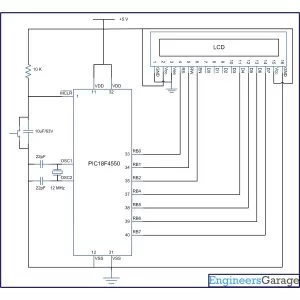# How to interface 16×2 LCD in 4-bit mode with PIC Microcontroller (PIC18F4550)

The 16×2 character LCD can work in two modes, namely, 8-bit and 4-bit. These modes basically correspond to the number of data pins used in interfacing LCD. 8-bit mode uses all the data lines and has been explained in LCD interfacing with PIC18F4550. In 4-bit mode, only four data pins of LCD are connected to the controller. This mode, thus, saves four pins of the controller unlike 8-bit mode. The configuration and display method of LCD in 4-bit mode has been explained here.

The 8-bit mode of LCD interfacing with PIC has been explained earlier. In the 4-bit mode the (8-bit) data/command is sent in nibble (four bits) format to LCD. The higher nibble is sent first followed by the lower nibble. In 4-bit mode only four data pins (D4-D7) of LCD are connected to the controller. The control pins (RS, RW and EN) are connected the same way as in 8-bit mode. The connections of LCD with PIC18F4550 are shown in the adjoining circuit diagram. Please note that here only PortB is used to connect data lines as well as control lines unlike in 8-bit mode. Refer LCD interfacing with PIC in 8-bit mode.

LCD is configured for 4-bit mode by sending appropriate instruction known as Function Set. The Function Set is hexadecimal instruction for LCD MPU unit which selects the working modes of LCD. The Function Set is given below along with its description.
 Instruction RS RW D7 D6 D5 D4 D3 D2 D1 D0 Function Set 0 0 0 0 1 DL N F – –

Description:
DL       –           Data Length
N         –           No. of Lines
F          –           Font

 Value DL N F 1 8 bit 2 lines 5×10 dots 0 4 bit 1 line 5×7 dots

Fig. 2: Bit Values to Configure LCD in 4-bit mode with PIC18F4550

According to the table, the value of Function Set for 4–bit mode will be [ 0010 0000 ] 0x20. The value of Function Set for the LCD configuration : 2 line (N=1), 5×7 dots (F=0) and 4-bit (DL=0) mode will be [ 0010 1000 ] 0x28.

It is important to note that when the power supply is given to LCD, it remains in 8-bit mode. In this state if 0x20 is sent, lower nibble will not be received by LCD because only four data lines (D4-D7) are connected, so 0x02 is sent instead of 0x20.

For more details on nibble arrangement and bit shifting, refer LCD 4-bit mode with AVR.

### Project Source Code

###

// Program to interface 16×2 LCD with PIC18F4550 Microcontroller using 4-bit mode

// Configuration bits
/* _CPUDIV_OSC1_PLL2_1L, // Divide clock by 2
_FOSC_HS_1H, // Select High Speed (HS) oscillator
_WDT_OFF_2H, // Watchdog Timer off
MCLRE_ON_3H // Master Clear on
*/

//LCD Control pins
#define rs LATA.F0
#define rw LATA.F1
#define en LATA.F2

//LCD Data pins
#define lcdport LATB

void lcd_ini();
void dis_cmd(unsigned char);
void dis_data(unsigned char);
void lcdcmd(unsigned char);
void lcddata(unsigned char);

void main(void)
{
unsigned char data0[]=”EngineersGarage”;
unsigned int i=0;
TRISB=0; // Configure Port B as output port
LATB=0;
lcd_ini(); // LCD initialization
while(data0[i]!=”)
{
dis_data(data0[i]);
Delay_ms(200);
i++;
}
}
void lcd_ini()
{
dis_cmd(0x02); // To initialize LCD in 4-bit mode.
dis_cmd(0x28); // To initialize LCD in 2 lines, 5×7 dots and 4bit mode.
dis_cmd(0x0C);
dis_cmd(0x06);
dis_cmd(0x80);
}

void dis_cmd(unsigned char cmd_value)
{
unsigned char cmd_value1;
cmd_value1 = (cmd_value & 0xF0); // Mask lower nibble because RB4-RB7 pins are being used
lcdcmd(cmd_value1); // Send to LCD
cmd_value1 = ((cmd_value<<4) & 0xF0); // Shift 4-bit and mask
lcdcmd(cmd_value1); // Send to LCD
}

void dis_data(unsigned char data_value)
{
unsigned char data_value1;
data_value1=(data_value&0xF0);
lcddata(data_value1);
data_value1=((data_value<<4)&0xF0);
lcddata(data_value1);
}

void lcdcmd(unsigned char cmdout)
{
lcdport=cmdout; //Send command to lcdport=PORTB
rs=0;
rw=0;
en=1;
Delay_ms(10);
en=0;
}

void lcddata(unsigned char dataout)
{
lcdport=dataout; //Send data to lcdport=PORTB
rs=1;
rw=0;
en=1;
Delay_ms(10);
en=0;
}

###

### Circuit Diagrams